Miscellaneous

Practical Geometry
Serial order wise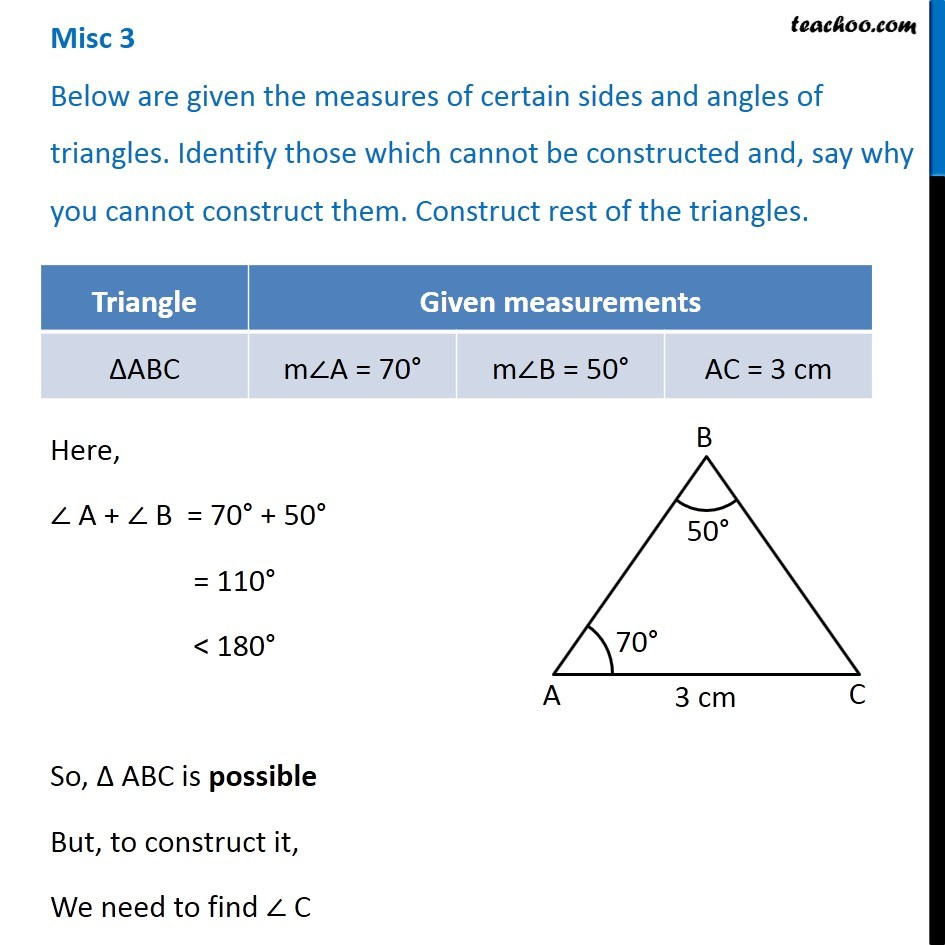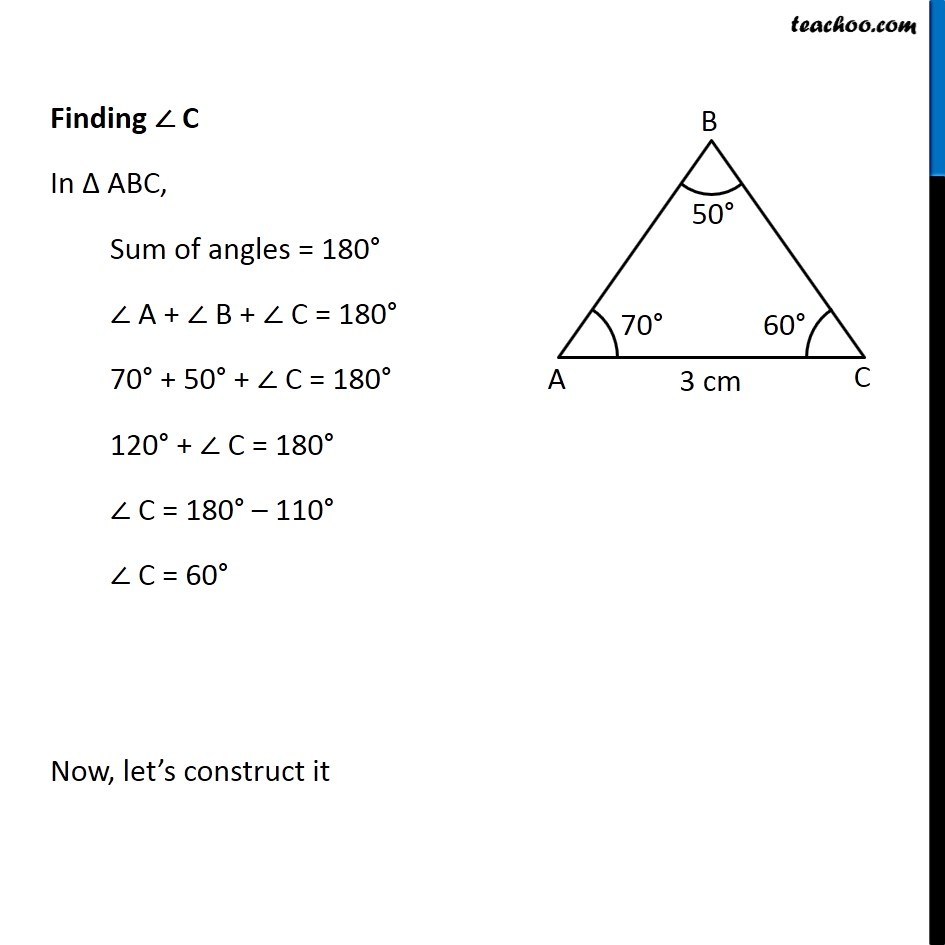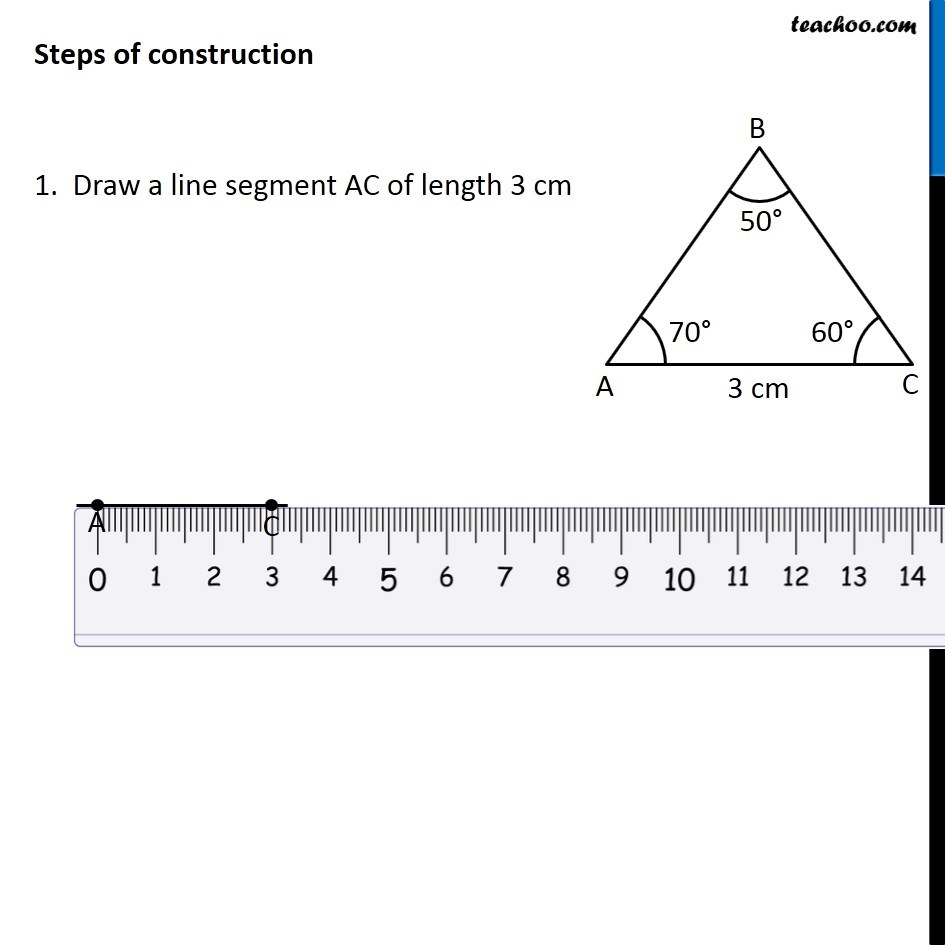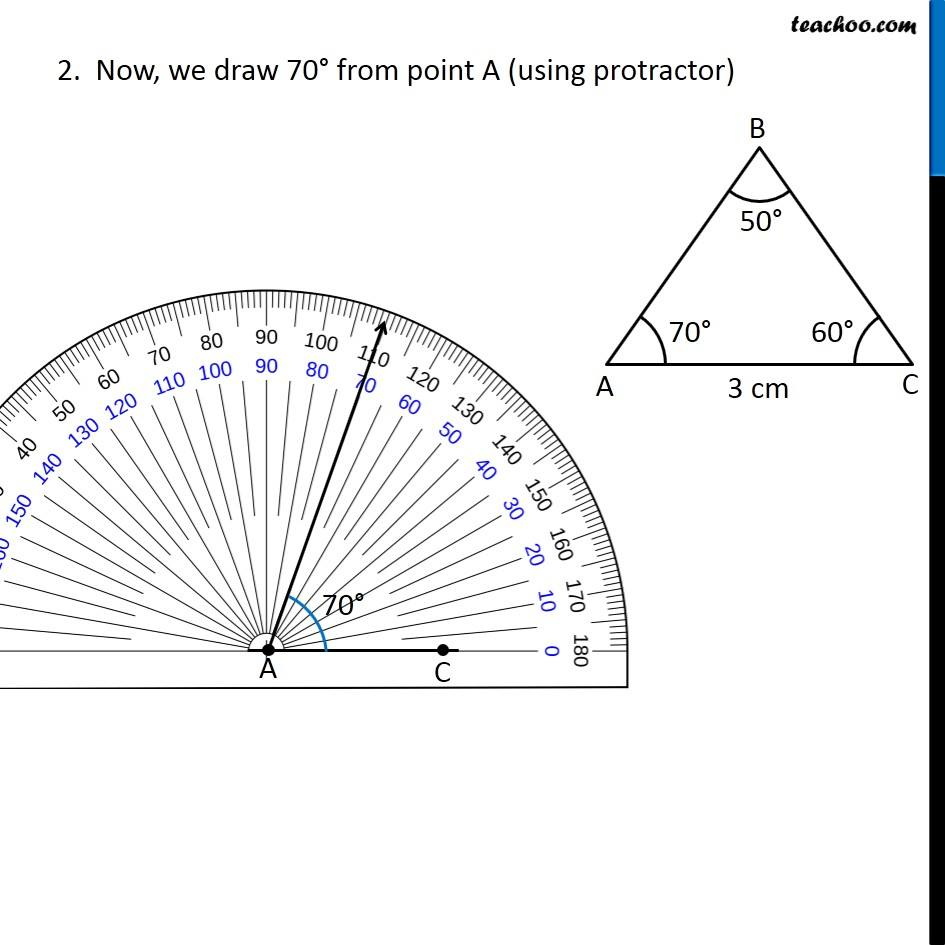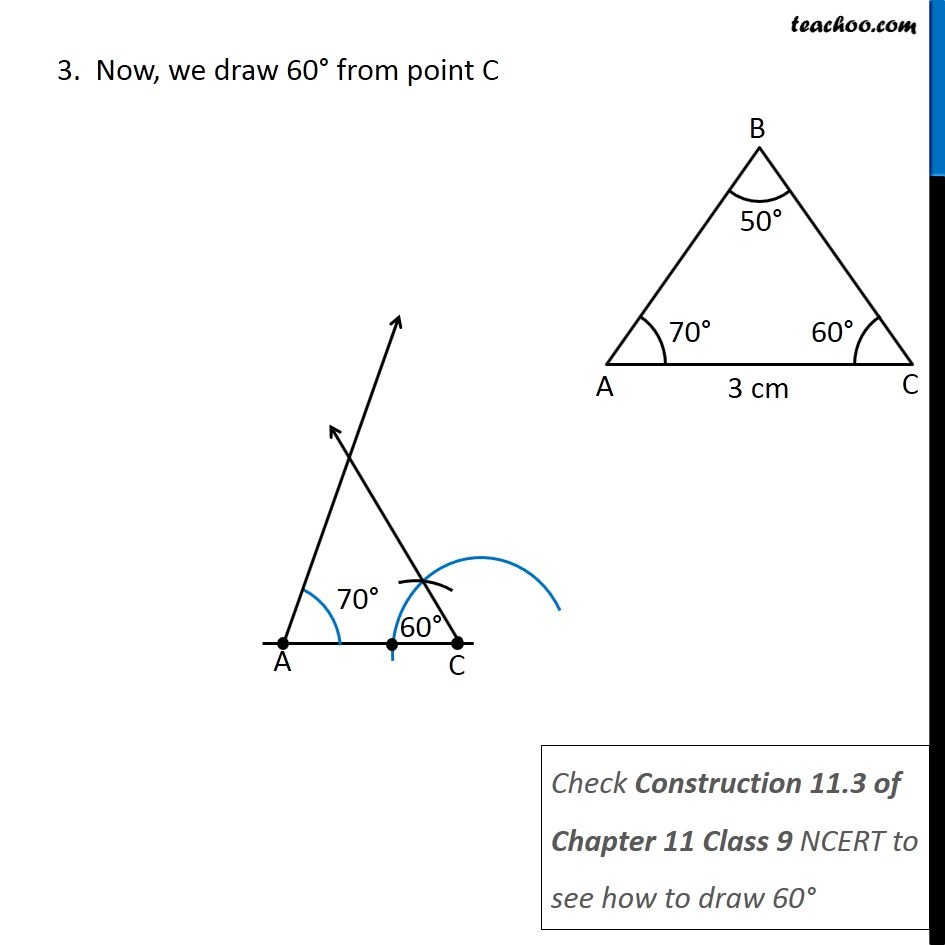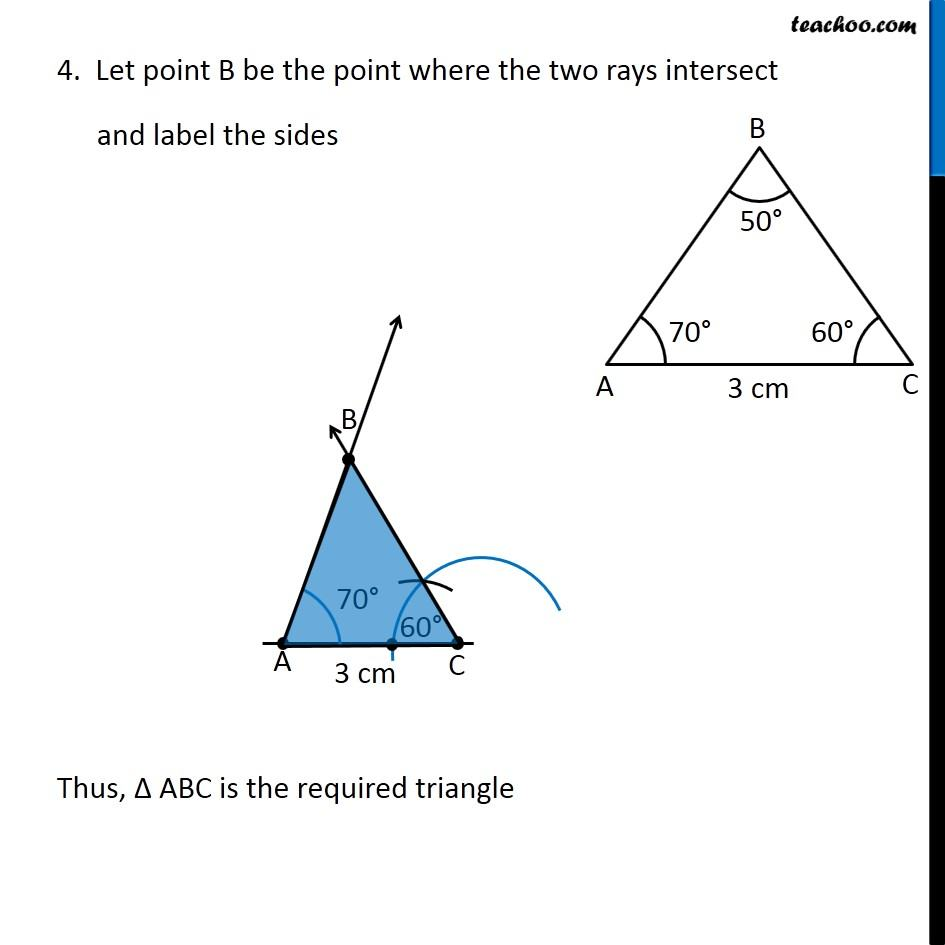Learn in your speed, with individual attention - Teachoo Maths 1-on-1 Class

### Transcript

Misc 3 Below are given the measures of certain sides and angles of triangles. Identify those which cannot be constructed and, say why you cannot construct them. Construct rest of the triangles. Here, ∠ A + ∠ B = 70° + 50° = 110° < 180° So, Δ ABC is possible But, to construct it, We need to find ∠ Finding ∠ C In Δ ABC, Sum of angles = 180° ∠ A + ∠ B + ∠ C = 180° 70° + 50° + ∠ C = 180° 120° + ∠ C = 180° ∠ C = 180° – 110° ∠ C = 60° Now, let’s construct it Steps of construction 1. Draw a line segment AC of length 3 cm 2. Now, we draw 70° from point A (using protractor) 3. Now, we draw 60° from point C Check Construction 11.3 of Chapter 11 Class 9 NCERT to see how to draw 60° 4. Let point B be the point where the two rays intersect and label the sides Thus, Δ ABC is the required triangle